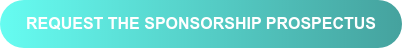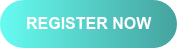<img height="1" width="1" style="display:none" alt="" src="https://www.facebook.com/tr?id=367542720414923&amp;ev=PageView&amp;noscript=1">

# Data & Analytics: Live, A/NZ

4-6 AUGUST, 2020 | DESKTOP, MOBILE & TABLET

## ENGAGE SENIOR DATA & ANALYTICS LEADERS AT THIS UNIQUE VIRTUAL EVENTtrue true true true true true true true true true true true true true true true true true true true true true true true true true true true true true true true true true true true true true true true true true true true true true true true true true true true true true true true true true true true true true true true true true true true true true true true true true true true true true true true true true true true true true true true true true true true true true true true true true true true true true true true true true true true true true true true true true true true true true true true true true true true true true true true true true true true true true true true true true true true true true true true true true true true true true true true true true true true true true true true

#### CLO APEX Seattle - Sponsor

+61 412118146

Terms & Conditions

The following Terms and Conditions (“Terms”) apply to you or your proxy in relation to your registration for this Corinium Global Intelligence (“Corinium”) offer. The definition of “registration” for purposes of these Terms will include all anyone who completes the above form. By entering your contact details, you agree to these Terms.